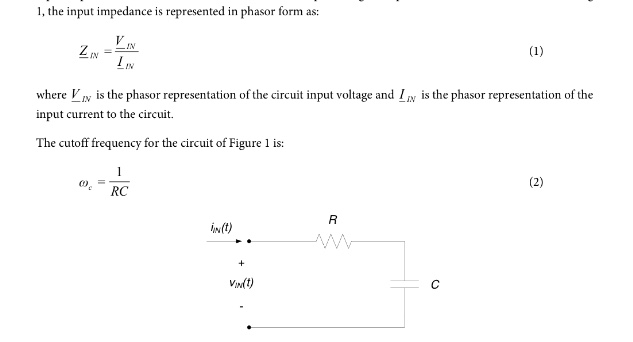# 1, Input impedance is represented in phasor from as:ZIN=VIN/IINwhere V_IN is the place representation of the circuit input voltage and IIN is the phasor representation of the input current to the circuit. The cutoff frequency for the circuit of Figure 1 is: Wc=1/RC a. Determine an expression for the input impedance of the circuit of Figure 1 in terms of R,C, and w. b. If R 100Ohm and C =1MuF,determine the cutoff frequency for the circuit. Also determine the input impedance for frequencies of:c. Check your low and high frequency results in part (b) relative to your expectations based on the capacitor’s low and high frequency behavior.

alot of explainarions are givenThis content is for Premium members only.